# 激活函数介绍

## sigmoid激活函数

### sigmoid激活函数的性质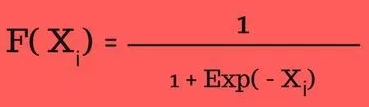• x: 输入
• float：表示浮点型数据
• exp：对其求指数
• f(x): 函数输出

• 非负： 如果输入数字大于或等于零；
• 非正： 如果输入数字小于或等于零；

### sigmoid激活函数的使用

• Sigmoid函数用于逻辑回归模型中的二进制分类。
• 在创建人造神经元时，Sigmoid函数用作激活函数。
• 在统计学中，S形函数图像是常见的累积分布函数。

### sigmoid激活函数python实现并画图

#### 实现代码

# Required Python Package
import numpy as np

def sigmoid(inputs):
"""
Calculate the sigmoid for the give inputs (array)
:param inputs:
:return:
"""
sigmoid_scores = [1 / float(1 + np.exp(- x)) for x in inputs]
return sigmoid_scores

sigmoid_inputs = [2, 3, 5, 6]
print "Sigmoid Function Output :: {}".format(sigmoid(sigmoid_inputs))


Sigmoid Function Output :: [0.8807970779778823, 0.9525741268224334, 0.9933071490757153, 0.9975273768433653]


#### 画图

# Required Python Packages
import numpy as np
import matplotlib.pyplot as plt

def sigmoid(inputs):
"""
Calculate the sigmoid for the give inputs (array)
:param inputs:
:return:
"""
sigmoid_scores = [1 / float(1 + np.exp(- x)) for x in inputs]
return sigmoid_scores

def line_graph(x, y, x_title, y_title):
"""
Draw line graph with x and y values
:param x:
:param y:
:param x_title:
:param y_title:
:return:
"""
plt.plot(x, y)
plt.xlabel(x_title)
plt.ylabel(y_title)
plt.show()

graph_x = range(0, 21)
graph_y = sigmoid(graph_x)

line_graph(graph_x, graph_y, "Inputs", "Sigmoid Scores")


Graph X readings: [0, 1, 2, 3, 4, 5, 6, 7, 8, 9, 10, 11, 12, 13, 14, 15, 16, 17, 18, 19, 20]

Graph Y readings: [0.5, 0.7310585786300049, 0.8807970779778823, 0.9525741268224334, 0.9820137900379085, 0.9933071490757153, 0.9975273768433653, 0.9990889488055994, 0.9996646498695336, 0.9998766054240137, 0.9999546021312976, 0.999983298578152, 0.9999938558253978, 0.999997739675702, 0.9999991684719722, 0.999999694097773, 0.9999998874648379, 0.9999999586006244, 0.9999999847700205, 0.9999999943972036, 0.9999999979388463]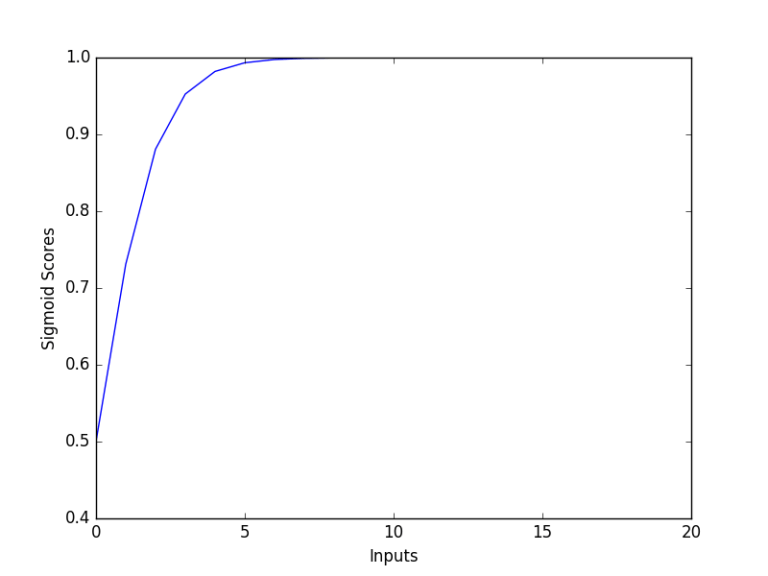## softmax激活函数

Softmax函数计算事件超过’n’个不同事件的概率分布。一般来说，这个函数将会计算每个目标类别在所有可能的目标类中的概率。计算出的概率将有助于确定给定输入的目标类别。

### softmax激活函数的性质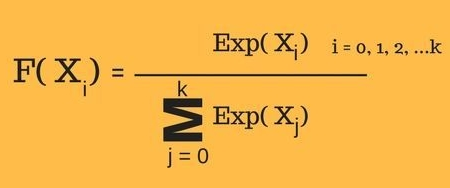• x: 输入
• exp：对其求指数
• f(x): 函数输出
从上述计算公式可以看出：
• 计算出的概率将在0到1的范围内。
• 所有概率的和等于1。

### softmax激活函数的使用

• 用于多重分类逻辑回归模型。
• 在构建神经网络中，在不同的层使用softmax函数。

### softmax激活函数python实现并画图

#### 实现代码

# Required Python Package
import numpy as np

def softmax(inputs):
"""
Calculate the softmax for the give inputs (array)
:param inputs:
:return:
"""
return np.exp(inputs) / float(sum(np.exp(inputs)))

softmax_inputs = [2, 3, 5, 6]
print "Softmax Function Output :: {}".format(softmax(softmax_inputs))


Softmax Function Output :: [ 0.01275478  0.03467109  0.25618664  0.69638749]


#### 画图

# Required Python Packages
import numpy as np
import matplotlib.pyplot as plt

def softmax(inputs):
"""
Calculate the softmax for the give inputs (array)
:param inputs:
:return:
"""
return np.exp(inputs) / float(sum(np.exp(inputs)))

def line_graph(x, y, x_title, y_title):
"""
Draw line graph with x and y values
:param x:
:param y:
:param x_title:
:param y_title:
:return:
"""
plt.plot(x, y)
plt.xlabel(x_title)
plt.ylabel(y_title)
plt.show()

graph_x = range(0, 21)
graph_y = softmax(graph_x)

line_graph(graph_x, graph_y, "Inputs", "Softmax Scores")


Graph X readings: [0, 1, 2, 3, 4, 5, 6, 7, 8, 9, 10, 11, 12, 13, 14, 15, 16, 17, 18, 19, 20]
Graph Y readings: [ 1.30289758e-09 3.54164282e-09 9.62718331e-09 2.61693975e-08 7.11357976e-08 1.93367146e-07 5.25626399e-07 1.42880069e-06 3.88388295e-06 1.05574884e-05 2.86982290e-05 7.80098744e-05 2.12052824e-04 5.76419338e-04 1.56687021e-03 4.25919483e-03 1.15776919e-02 3.14714295e-02 8.55482149e-02 2.32544158e-01 6.32120559e-01]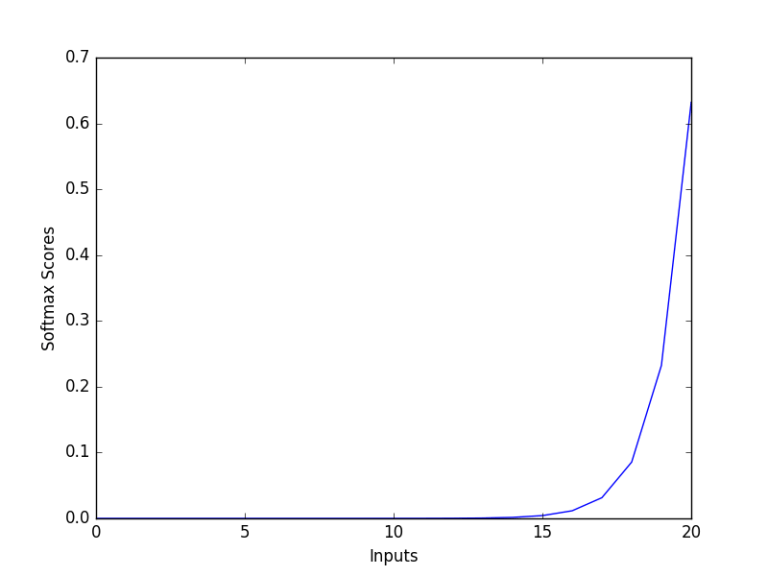# 多类分类及多标签分类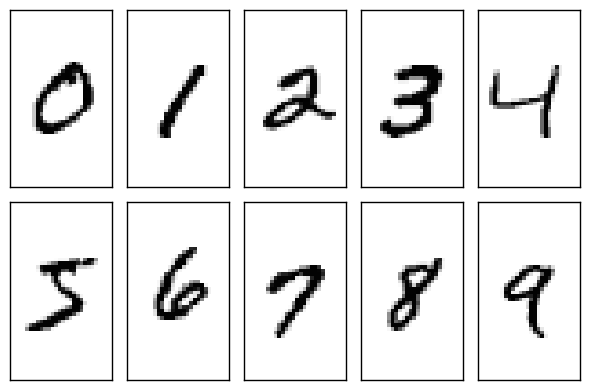# 使用softmax和sigmoid激活函数来做多类分类和多标签分类

## softmax激活函数应用于多类分类

tf.argmax(tf.softmax(logits))


## sigmoid激活函数应用于多标签分类

sigmoid一般不用来做多类分类，而是用来做二分类，它是将一个标量数字转换到[0,1]之间，如果大于一个概率阈值(一般是0.5)，则认为属于某个类别，否则不属于某个类别。这一属性使得其适合应用于多标签分类之中，在多标签分类中，大多使用binary_crossentropy损失函数。它是将一个标量数字转换到[0,1]之间，如果大于一个概率阈值(一般是0.5)，则认为属于某个类别。本质上其实就是针对logits中每个分类计算的结果分别作用一个sigmoid分类器，分别判定样本是否属于某个类别同样假设，神经网络模型最后的输出是这样一个向量logits=[1,2,3,4,5,6,7,8,9,10], 就是神经网络最终的全连接的输出。这里假设总共有10个分类。通过：

tf.sigmoid(logits)


sigmoid应该会将logits中每个数字都变成[0,1]之间的概率值，假设结果为[0.01, 0.05, 0.4, 0.6, 0.3, 0.1, 0.5, 0.4, 0.06, 0.8], 然后设置一个概率阈值，比如0.3，如果概率值大于0.3，则判定类别符合，那么该输入样本则会被判定为类别3、类别4、类别5、类别7及类别8。即一个样本具有多个标签。

07-056009
09-12385
11-12204
12-04242
10-09448
09-012551
07-04995
05-04302
02-083002
04-301616
07-241万+
11-232301
10-12391
11-141145
10-011万+
11-231719
05-142678
04-261139

### “相关推荐”对你有帮助么？

•非常没帮助
•没帮助
•一般
•有帮助
•非常有帮助被折叠的  条评论 为什么被折叠?到【灌水乐园】发言uncle_ll

¥2 ¥4 ¥6 ¥10 ¥20余额支付 (余额：-- )扫码支付获取中扫码支付点击重新获取扫码支付1.余额是钱包充值的虚拟货币，按照1:1的比例进行支付金额的抵扣。
2.余额无法直接购买下载，可以购买VIP、C币套餐、付费专栏及课程。余额充值